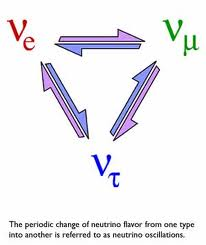# A Neutrino’s Mass: Challenging Physicists and….Mathematicians?Some puzzles exist beyond the confinement of pure mathematical thought and forge their way into the realm of physical reality.   In 1930, the theoretical physicist Wolfgang Pauli brought one such conundrum to light.  In a letter to famed physicist Lise Meitner, Pauli postulated the existence of a neutrally charged particle with mass less than one percent of a proton to explain underlying phenomena in beta decay.   Neutrinos, as they are now called, come in three flavors ; electron, muon, and tau.

By the Standard Model of Particle Physics (a theory that seeks unification through properties of sub-atomic particles), neutrinos were assumed to be massless.  However, contrary to theory, experimental results yielded that neutrinos have mass.    The resulting question is: What is the measurement of a neutrino’s mass?  No one knows.

Associate professor of physics, Joseph Formaggio of MIT has built his career around the problem.  In an article written by Anne Trafton on the MIT News site entitled, “On the hunt for neutrinos” (found here: http://ow.ly/mPScf) ,   Trafton quotes Formaggio explaining the significance of the research:

“The SNO experiments revealed that there are three ‘flavors’ of neutrino that can morph from one to the other. Those experiments, basically put the nail in the coffin and said that neutrinos change flavors, so they must have mass,…. It was a big paradigm shift in thinking about neutrinos, because the Standard Model of particle physics wants neutrinos to be massless, and the fact that they’re not means we don’t understand it at some very deep level.”Trafton later adds, “By measuring the energy of the electron released during the decay, physicists hope to be able to calculate the mass of the neutrino — an approach based on Einstein’s E=mc2 equation.”

With all of this in mind, what do mathematicians think about the problem?  Is it intuitive to assume that there has to be  a mathematical tool that could deduce the measurement of a neutrino’s mass rigoursly without much experimental validation? If so, what tool?  Since the particle has mass, classical mechanics guarantees that it has a gravitational field.  However, the gravitational influence of a neutrino is so faint that it has never been detected with current experimental approaches.   Could the answer be found in a geometrical or topological relationship waiting to be discovered?

An internet search will give you a list of theories that propose a measurement.  However none have been validated with experimentation.   Furthermore, it would take an ingenious approach to derive it with precise mathematical rigor that extends or augments the tools of theoretical physics.  A challenge that I do not believe is out of reach for someone with a little creativity and mathematical maturity coupled with a good grasp of physical law.  Maybe that someone is you.